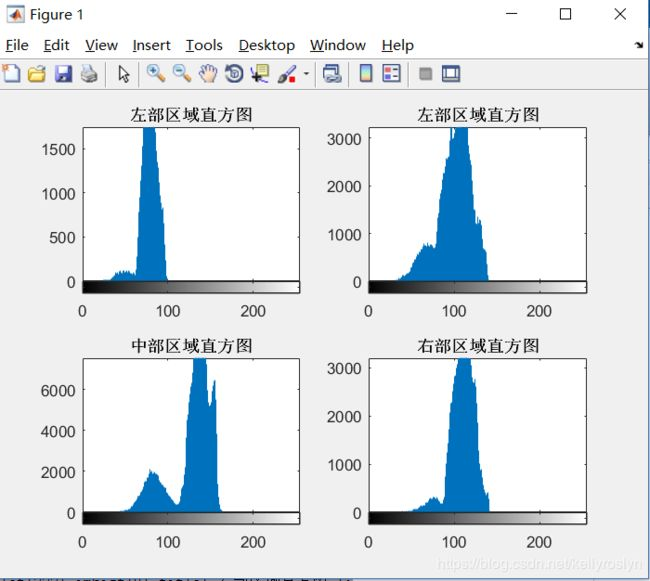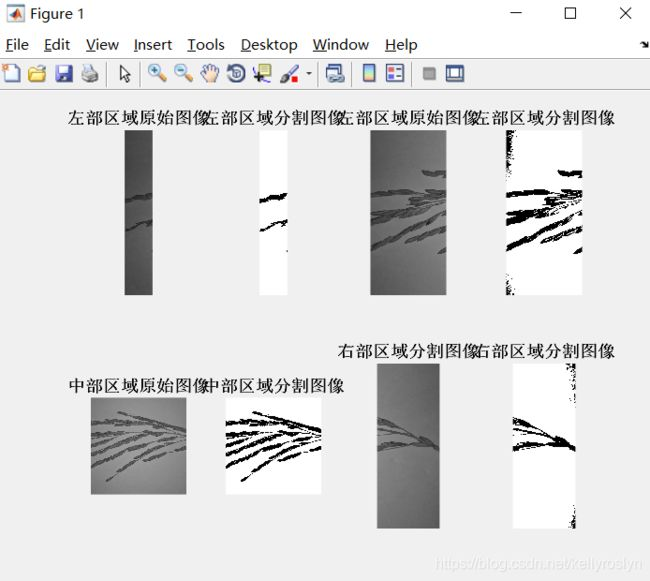• 1.如何在一figure里面画多个子图。%subplot(x,y,n)x表示显示的行数，y表示列数，n表示第几幅图片figure,subplot(221),imhist(P),title('左部区域直方图'); % define figuresubplot(222),imhist(U),title('左部区域...

此博文仅为个人学习笔记，仅供个人使用。

1.如何在一个figure里面画多个子图。

%subplot(x,y,n)x表示显示的行数，y表示列数，n表示第几幅图片

figure,subplot(221),imhist(P),title('左部区域直方图'); % define figure

subplot(222),imhist(U),title('左部区域直方图');

subplot(223),imhist(D),title('中部区域直方图');

subplot(224),imhist(T),title('右部区域直方图');%2行4列的图片，一共8幅图。

figure,subplot(241),imshow(P),title('左部区域原始图像');%321是3行2列矩阵第1幅图

subplot(242),imshow(L0),title('左部区域分割图像');

subplot(243),imshow(U),title('左部区域原始图像');%321是3行2列矩阵第1幅图

subplot(244),imshow(L1),title('左部区域分割图像');

subplot(245),imshow(D),title('中部区域原始图像');

subplot(246),imshow(L2),title('中部区域分割图像');

subplot(247),imshow(T),title('右部区域分割图像');

subplot(248),imshow(L3),title('右部区域分割图像');2.多个figure画多个子图

figure(1); % 第一个子图

plot(...);

figure(2); % 第二个子图

plot(...);

figure(3);

plot(...);

figure(4);

plot(...);

展开全文• Matlab中的程序有以下三种类型,现举例如下：(1)f=@(x)x^2+x+1(2)syms x;f=2*x^3-x^2+1或syms x;f=inline('2*x^3-x^2+1')(3)建立M文件function f=fun(x)f=x^2-2*x+1对于你的问题，有多种解决方法，现举一例(是我认为...Matlab中的程序有以下三种类型,现举例如下：

(1)f=@(x)x^2+x+1

(2)syms x;f=2*x^3-x^2+1

syms x;f=inline('2*x^3-x^2+1')

(3)建立M文件

function f=fun(x)

f=x^2-2*x+1

对于你的问题，有多种解决方法，现举一例(是我认为最好的解决办法)

(1)建立M文件，在其中输入如下内容：

function f=fun(x,i)

%x,i均位向量。

f=zeros(length(i),length(x));%预分配变量空间

for k=1:length(i)

f(k,:)=x.^i   %相当于建立一个含有两个变量的函数。

end

在调用时，如果你需要计算x=[1:10];i=1:2;时f的函数值。可以在MATLAB命令窗口中输入如下命令：

x=[1:5];i=1:2;

f=fun(x,i)

运行所得结果为：

f=

1 2 3 4 5

1 4 9 16 25

当然，此时，若只需求某一个数的某个次方也行。

例如：要求6^3,在MATLAB命令窗口中输入

y=fun(4,3)

得到结果为

y=

64

其他，依次类推。

◆◆

评论读取中....

请登录后再发表评论!

◆◆

修改失败，请稍后尝试

展开全文• matlab画传递函数曲线

千次阅读 2021-05-07 07:26:34
如何matlab画开环传递函数的奈奎斯特图用MATLAB做出奈奎斯特曲线图%k=10k=10;d=conv(,conv([0.51],[0.21]));Gs=tf(k,d);nyquist(Gs);稳定性可根据奈奎斯特判据判定：开环系怎样用MATLAB输入一传递函数以G(s)=...

如何matlab画开环传递函数的奈奎斯特图

用MATLAB做出奈奎斯特曲线图%k=10k=10;d=conv(,conv([0.51],[0.21]));Gs=tf(k,d);nyquist(Gs);稳定性可根据奈奎斯特判据判定：开环系

怎样用MATLAB输入一个传递函数

以G(s)=10/s(s+1)(s^2/4+1)为例这种传递函数是零极点描述形式,因而要使用sys=zpk(z,p,k)的命令形式其中,z为传递函数的零点向量[z1,z2,z3,...],描述形式为(

matlab画函数曲线问题

symsptsum=M=[1,2;3,4;2,3;2,3];fort=0.2:0.1:1;p=[1,t,t.^2,t.^3]*M;sum=[sum;p];endsum(1:1,:)=[]A=s

matlab开环传递函数与闭环传递函数的应用场合

求系统的响应,应该用闭环传递函数；绘制根轨迹、伯德图,应该用开环传递函数.这道题目给出来的就是典型二阶系统的闭环传递函数.

MATLAB画函数曲线

clc;x=0:0.1:15;y=0.4045879*cosh(0.79304*x).*cos(0.79304*x)+0.3530811.*sinh(0.79304*x).*sin(0.79304*x

关于MATLAB传递函数的仿真

但看有的论文系统辨识了,不知道辨识是为了得到什么呀?是为了得到合适的权值和阀值么?但在线调整不是能改变么?高手解惑呀?谢谢不能改再问：.......

如何利用matlab画曲线

法1ezplot例子ezplot('x^2',[0,1])ezplot('exp(-x)')%这里exp(-x)表示e的-x次方.即e^(-x)法2fplot例子fplot('x^2',[0,1])f

在matlab中,零输入响应是什么函数?比如给出系统开环传递函数,求其零输入响应曲线

换路后,电路中无独立的激励电源,仅由储能元件的初始储能维持的响应.也可以表述为,由储能元件的初始储能的作用在电路中产生的响应称为零输入响应(Zero-inputresponse).零输入响应是系统微分

matlab里传递函数的问题

这个函数表示的是微分方程：dy1/dt=y2dy2/dt=-(B/J)*y2+(1/J)*u在零初始条件下进行拉氏变换,以y1作为系统的总输出,把第1个方程代入第2个,稍加整理得到s^2*y1+(B/

matlab 传递函数问题

传递函数：G(S)=ωn^2/(S^2+2*ζ*ωn*S+ωn^2)

怎样用Matlab画曲线

[x,y,z]=meshgrid(linspace(-1,1));v=x.^2+y.^2+z.^2-1;[xx,zz]=meshgrid(linspace(-2,1));yy=1-xx;h=conto

z传递函数、s传递函数在matlab中如何转换?

从离散转换为连续在控制工程里有时会用得到,因为虽然控制器是离散的但被控对象是连续的,所以d2c是控制工具箱函数,以下例子中tf,tfdata都是控制工具箱的函数以下是Z变换到S变换dsys=tf([1

求这个MATLAB传递函数的单位阶跃响应曲线

对于正常的系统传函,求阶跃响应很简单,一般可以用类似下面的代码：s=tf('s');Gc=2*(0.2*s+1)*(0.1*s+1)/(0.2*s);step(Gc)但上述代码会出错：

matlab画平滑的曲线

用差值m2=[1.24001.42002.69005.13008.520012.750017.390022.200027.410028.670032.3300];nta=[0.25950.28400.

matlab画积分函数曲线

这个问题和另一个问题(编号2051722037141864067)基本相同,但与那个问题相比,又多了一处错误：f1=integral(@(v)f0(v,x),0,inf); f2=array

已知传递函数,求幅频响应?matlab

可以这样,a=;b=[-101];%输入系统的系数矩阵[X,w]=freqz(b,a);%求取系统频率响应plot(w/pi,abs(X));%画解卷绕后的幅值响应grid;不知道你明白没有

如何用matlab画开环传递函数的伯德图

在命令行输入helpbode,然后看最下面一行,点docbode就是例子啊.要会用help哦,很有用的命令.

matlab由函数画曲线

>> T=8:.5:35;,D=9000:20000;[DD,TT]=meshgrid(D,T);K=290.0503.*TT.^(-0.1349).*DD.^(0.3350);

matlab怎样画三维曲线

http://wenku.baidu.com/view/cabfa7eae009581b6bd9ebfc.html,这个文库文件可能有你想要的结果!

展开全文• clear;clc;%% parameter to adjustss = 0;ds = 0.01;es = pi/2;sb = 0;db = 1e-2;eb = pi/2;%% calculate and plot% calculate the first functionn = 1;for sita = ss:ds:esbeta = sb:db:eb;tem = cos(beta)*sin(si...

clear;clc;

ss = 0;

ds = 0.01;

es = pi/2;

sb = 0;

db = 1e-2;

eb = pi/2;

%% calculate and plot

% calculate the first function

n = 1;

for sita = ss:ds:es

beta = sb:db:eb;

tem = cos(beta)*sin(sita) .* sqrt( 1 - cos(2*beta)*sin(2*sita) );

y1(n) = sum( tem ) * db;

n = n+1;

end

sita = (180/pi) * (ss:ds:es);

subplot(2,2,1);

plot( sita, y1);

xlabel( 'cos(beta)*sin(sita) .* sqrt( 1 - cos(2*beta)*sin(2*sita) )');

title('first function');

% calculate the second function

n = 1;

[ fa, gama ] = meshgrid(sb:db:eb, sb:db:eb );

for sita = ss:ds:es

tem = sqrt( 1 - cos(2*fa) .* ( 1 - sin( 2*sita ) * cos( 2*gama ) ) );

y2(n) = sum( sum( tem ) ) * db^2;

n = n+1;

end

sita = (180/pi) * (ss:ds:es);

subplot(2,2,2);

plot( sita, y2);

xlabel( 'sqrt( 1 - cos(2*fa) .* ( 1 - sin( 2*sita ) * cos( 2*gama ) ) )');

title('second function');

%% plot both

subplot(2,2,3);

plot( sita, y1); hold on

plot( sita, y2); hold off

title('both function');，

展开全文• 如何用matlab画出函数曲线

千次阅读 2021-04-19 00:38:11
使用matlab绘制函数曲2113线的方法和操作步5261骤如下：1、首先，使用matlabc打开命令行窗口，然后4102直接输入相1653关内容，如下图所示。2、其次，完成上述步骤后，请根据实际情况设置图示代码，如下图所示。3、...
• 点击查看怎么用matlab画已知函数表达式的一个函数图像？函数比较复杂的……具体信息答：要具体哦，如果一个自变量，函数图像就是曲线，用plot 如果是2个自变量，函数图像就是曲面了，如f=3*exp(-x-4*y),可以用以下...
• dao可用meshgrid和mesh函数来绘制曲面，内举例子如下，容具体用法可参加helpmesh等。:lol[x,y]=meshgrid(-1:.05:1);z=x.^2+sin(y);mesh(x,y,z)，曲线还是曲面，曲线的话用plot3函数，曲面是mesh，还有surf,surfl等...
• matlab函数多个输出

千次阅读 2021-04-18 04:17:28
matlab函数多个输出可以输出多个是不是因为你在函数中没有给输出变量赋值？给一个例子function [a,b,c]=test1(input)if input==1a=ones(2);b=ones(3);c=ones(4);elsea=zeros(2);b=zeros(3);c=zeros(4);end函数调用&...
• matlab程序 在同一图中绘制两个函数,这两个函数分别是： , ,,matlab怎样同时出两个函数图用matlab程序 在同一图中绘制两个函数,这两个函数分别是： , ,其...,matlab怎样同时出两个函数图匿名网友:这个函数和...
• 分段函数如下：对该函数绘图，首先应该利用matlab建一个函数ff(x),如下：functionY=ff(X)ifX<0;Y=sin(X);elseif(X>=0&&X<10);Y=X.^2*cos(X)/100;elseY=sin(X).*X;endendmatlab中二维绘...
• 问题描述：Matlab多参数函数小弟想用三变量p、q、s拟合出一条关于y的曲线,已知p、q、s各自关于y的关系(都是二次函数),该如何实现呢?大侠,好人做到底,送佛送上天,现在我告诉您y=x(1)*p.^2+x(2)*p+x(3)*q.^2+x(4)*q+...
• 一个抄m文件中定义了多个函数的话，这些函2113数只能在这个5261m文件中的函数4102中调用。m文件基本上就是函数，例如第二个m文件函数名为 function mode(a,b) 则第一个m调用时写mode(a,b)即可function 函数名1653按...
• matlab帮助系统中的说明：FunctionsThe main difference between a script and a function is thata function accepts input from and returns output to its caller, whereasscripts do not. You define MATLAB ...
• 如何用Matlab画二元函数？

千次阅读 2021-04-18 06:07:49
1、首先打开matlab。2、在 matlab 当前目录空2113间右键5261。41023、然后点击 new -> M-File。4、然后将文件命令为hello.m。5、然后双击该文1653件，输入[Rm dm]=meshgrid(15:5:50,1:10);6、然后添加f=0.034488*...
• 可以输出多个2113是不是5261因为你在函数中没有4102给输1653出变量赋值？专给一个例子属function[a,b,c]=test1(input)ifinput==1a=ones(2);b=ones(3);c=ones(4);elsea=zeros(2);b=zeros(3);c=zeros(4);end函数调用&...
• 1.把当前窗口分成四区域，用不同的颜色和线条分别绘制sin(x),cos(x),e^x,log(x)的函数图形，并加入文字标示和网格。clearclcx=-2*pi:0.01:2*pi;y1=sin(x);y2=cos(x);y3=exp(x);t=0:0.01:20;y4=log(t);subplot(221)...
• 一、plot 函数绘制多个图形、 二、legend 函数标注图形、 三、图形修饰、matlab plot legend
• [2018年最新整理]matlab画复数函数图象和黎曼面用matlab画复数函数图像探究及体会黄清华 学号1207020025(物理一班)戴刘江 学号1207020013(物理一班)摘要：本文主要写了小组自学用matlab画黎曼面的一些体会，并给出了...
• nargin： number of function arguments，即函数输入参数数。只要你的函数重载逻辑没有出错，那么就可以通过查看nargin的数值来实现你说的设置缺省值等功能。看下nargin的帮助就知道了。使用方法nargin是用来判断...
• x=0.001:0.1:10;a=1;f=(1/a)*exp(-x/a);F=1-exp(-x/a);plot(x,f,'r',x,F,'b') %...matlab在画图时其实就是bai采用离散点来完成du的如果zhi您想让x当做横坐标的话可dao以这样plot(ones(20,1)*[300 400],linspace(-1...
• Matlab中一个函数调用另外一个函数的操作步骤作者:小安 来源:PC下载网时间:2019-11-18 13:27:35最近很伙伴才刚刚安装入手Matlab这款软件，而本节就重点介绍了关于Matlab中一个函数调用另外一个函数的操作步骤，还...函数调用matlab
• matlab中可以利用三维数62616964757a686964616fe78988e69d8331333361323032据等值面绘制函数isosurface来绘制三维隐函数的图像三维隐函数可以描述，为方程 f(x,y,z)=0;如果我们定义函数v=f(x,y,z)在一定的下x，y，...
• 函数想必大家都不陌生，有时候我们需要函数的图形，但是复杂的函数我们出来花费时间太，下面我来教大家用matlab画函数图像。工具/材料电脑matlab软件操作方法01先下载安装这软件，然后打开，先来教大家...
• 《用matlab画出余弦函数的傅里叶变换图像》由会员分享，可在线阅读，更相关《用matlab画出余弦函数的傅里叶变换图像(3页珍藏版)》请在人人文库网上搜索。1、用matlab画函数和余弦函数的傅里叶变换一、由狄拉克...
• Matlab在一个图形窗口里画多个图形的详细教程作者:佚名 来源:PC下载网时间:2019-11-18 13:35:08对于Matlab软件各位应该还有不懂的地方，比如说想在一个图形窗口里画多个图形，却不了解相关的操作，而今天小编就带来...
• 本文收集整理关于matlab定义一函数的相关议题，使用内容导航快速到达。内容导航：Q1：matlab怎么建立自定义函数自定义函数的格式：function 函数名(参数)函数表达式end并保存为 函数名.m 的文件(函数名由英文和...
• matlab函数返回多个值--样例

千次阅读 2021-04-18 03:25:33
matlab 函数返回多个值--样例发布时间：2018-02-14 19:52,浏览次数：2062, 标签：matlab目录一、matlab函数文件返回多个值写法二、简单的例子三、 结果截图一、matlab函数文件--返回多个值--写法为：1....matlab函数结果
• 对于初学者来说，学习matlab的时候编写一些程序难度确实比较大，因为初学者对于很多函数的使用都不是很熟悉。为了帮助初学者学习matlab，这里给出一些函数波形程序，希望对大家有所帮助。那么，matlab怎么冲激函数...
• 一些选择：添加一参数以指定控制台的详细输出,但默认情况下将其设置为false：function [ A, B, C ] = test(x, y, z, verbose)if nargin = 3verbose = false;end;A=2*x;B=2*y;C=2*z;if verbosefprintf('A = %f\nB =...matlab函数结果
• 我只知道运算时要用到数组运行，但不知要加到哪里，所以用了循环。比如：x=0:0.01*pi:pi;y=sin(x).*cos(x);plot(x,y);这样才行。但你这道题，我不知要把点加哪。不好意思，只能用到循环了www.mh......

matlab画多个函数matlab 订阅